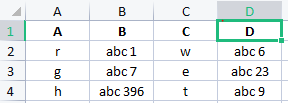# How to replace row values of specific column in excel?

Hi Community,I want to know how to replace column B and column D with only numeric values.
E.g . Output for Column B is : 1 Output for Column D is : 6
7 23
396 9
The format is fixed only numeric values are dynamic.

I know how to replace single value : String.Replace(“abc”,“”)
But for multiple rows in excel for specific column I’m not able build the logic.

Any help would be appreciated.
Thanks in AdvanceYou can use regex to extract the numeric values from the columns & replace the data with the extracted value.

RegEx = \d+

Hope this helps,
Best Regards.

Hi
Can you tell the steps how to do it ?

@Vaishnav_Tej

Read the sheet as datatable & execute this query in Invoke Code to update ColumnB & ColumnD:

``````dt.AsEnumerable.ToList.ForEach(Sub(row)

row(“B”) = if(System.Text.RegularExpressions.Regex.IsMatch(row(“B”).ToString, "\d"), System.Text.RegularExpressions.Regex.Match(inputString, "\d+").Value, row(“B”).ToString)

row(“D”) = if(System.Text.RegularExpressions.Regex.IsMatch(row(“D”).ToString, "\d"), System.Text.RegularExpressions.Regex.Match(inputString, "\d+").Value, row(“D”).ToString)

End Sub)
``````

This query will first check in both ColumnB & ColumnD for a string for the presence of any numeric character, if so, it will extract the number & update the same in these columns.

Hope this helps,
Best Regards.

1 Like

Excel Application Scope > Read range to DT … call it excellDT

Activity: for each row in datatable
click on “for each row in datatable” and for index, click ctrl+k create new variable called index

for column B … (I’m assuming you don’t have headers? )
inside of for each

activity: matches
input: CurrentRow(1).tostring
Pattern: \d+
output (press ctrrl+K and save): OnlyNumbersB

activity Write Cell
“B”+Index+1.ToString
value: OnlyNumbersB(0).ToString or if you want as integer cint(OnlyNumbersB(0).ToString)

repeat same for column D

You could try the following Linq Statement:

``````(From row In dtTest.AsEnumerable()
Let filteredB = New String(row.Field(Of String)("B").Where(Function(c) Char.IsDigit(c)).ToArray())
Let filteredD = New String(row.Field(Of String)("D").Where(Function(c) Char.IsDigit(c)).ToArray())
Select dtTest.Clone().Rows.Add(row.Field(Of String)("A"), filteredB, row.Field(Of String)("C"), filteredD)).CopyToDataTable()
``````

Replace “dtTest” with you datatable name and assign it to a datatable variable(Use the assign activity in UiPath, left side = variable(e.g. dtTestTest) and right side = Linq Statement above. I guess your columns are of type string.
Try it, may need some modification based on needs.

Keep in mind that the above statement only works for whole numbers
e.g. “abc 17” would turn out to “17”.
and “abc 17.1” would turn out to “171”.

This is a “ugly” way to take “.” and “,” in consideration(if a word contains “,” or “.” e.g. “ab,c 17” output = “,17”):

``````(From row In dtTest.AsEnumerable()
Let filteredB = New String(row.Field(Of String)("B").Where(Function(c) Char.IsDigit(c) OrElse c = "." OrElse c = "," ).ToArray())
Let filteredD = New String(row.Field(Of String)("D").Where(Function(c) Char.IsDigit(c) OrElse c = "." OrElse c = "," ).ToArray())
Select dtTest.Clone().Rows.Add(row.Field(Of String)("A"), filteredB, row.Field(Of String)("C"), filteredD)).CopyToDataTable()
``````

There is also a posibility to use the “IsNumeric” function and other variants.

@Vaishnav_Tej

Your replace function is correct…what you need to do is to perform this action in a for each row in datatable or for each excel row activities

Inside ethat use `curretrow("Column") = currentrow("Column").ToString.Replace("abc","")`

If abc is not constant then use regex as suggested by @arjunshenoy

Cheers

2 Likes